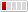0 Replies Latest reply on Jul 7, 2006 8:30 PM by Alex Levine

# issue finding entity with EmbeddedId using EntityManager.finI have a table similar to the following
MY_TABLE(
COL1 VARCHAR,
COL2 VARCHAR,
COL3 VARCHAR,
COL4 VARCHAR,
PRIMARY KEY(COL1, COL2, COL3)
)

where there is a composite primary key mad up of columns COL1, COL2, COL3.

I have the following class structure (none, some non-pertinent code is left out)

@Embeddable
public class MyTablePK implements Serializable
{
public MyTablePK(String COL1, String COL2, String COL3)
{...}

public String getCOL1();
public String setCOL1(String s);

public String getCOL2();
public String setCOL2(String s);

public String getCOL3();
public String setCOL3(String s);

public equals(Object o);

public int hashCode();
}

@Entity
@Table(name = "MY_TABLE")
public class MyTable
{
@EmbeddedId
public MyTablePK getID();

protected void setID(MyTablePK id)

@Column(name = "COL4")
public getCOL4();
public setCOL4(String COL4);
}

I can create one of these and persist it using em.persist and that works fine. Now assume I know I have a row in the DB where COL1 = 'val1', COL2 = 'val2', and COL3 = 'val3'. When I try to then create a PK with those valuse and then use the EnittyManager to find it using the find method it returns null:

MyTablePK pk = new MyTablePK("val1", "val2", "val3");
// return null
MyTable mt = entityManager.find(MyTable.class, pk);

However when I use a Query I can find the object in the following manner:
MyTable mt = entityManager.createQuery("from MyTable m where m.ID.COL1 = ?1 and m.ID.COL2 = ?2 and m.ID.COL3 = ?3")
.setParameter(1, "val1")
.setParameter(2, "val2")
.setParameter(3, "val3).getSingleResult();

the entity is returned properly. Then if I take the returned entity and do the following it works:

// now returns proper value
MyTable mt2 = entityManager.find(MyTable.class, mt.getID());

I have compared the primary key that I manually composed and the one that is returned from mt.getID and they appear to be the same ( when comparing them with the equals method it returns true and their hash codes are the same), so how come the manually created one does not work in the find method?? Is this a bug or am I doing something wrong??